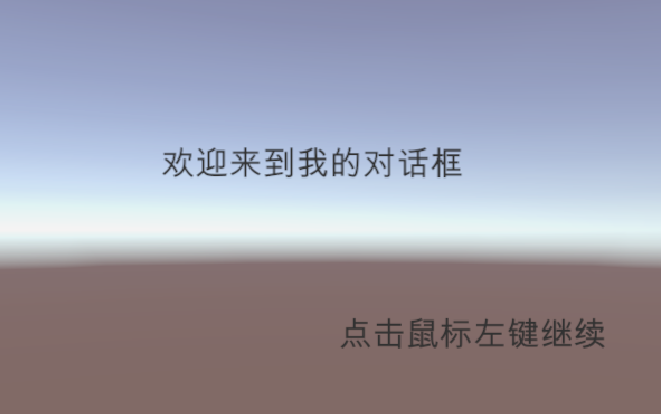# unity制作对话框public float charsPerSecond = 0.2f;//打字时间间隔
private string[] words = {"欢迎来到我的对话框","接下来给大家看一下","快要结束了哦" };
//保存需要显示的文字 我这里面是三句
public int strindex = 0;//控制语句

private bool isActive = false;
private float timer;//计时器
private Text myText;
public int currentPos = 0;//当前打字位置

void Start()
{
timer = 0;
isActive = true;
myText = GetComponent<Text>();
}
void Update()
{
OnStartWriter();
if (Input.GetMouseButtonDown(0))
{
strindex++;//下一句
isActive = true;
if (strindex >= 2)//防止超出数组的长度报错
{
strindex = 2;
}
}
}

public void StartEffect()
{
isActive = true;
}
/// 执行打字任务
void OnStartWriter()
{
if (isActive)
{
timer += Time.deltaTime;
if (timer >= charsPerSecond)
{//判断计时器时间是否到达
timer = 0;
currentPos++;
myText.text = words[strindex].Substring(0, currentPos);//刷新文本显示内容

if (currentPos >= words[strindex].Length)
{
OnFinish();
}
}

}
}
/// 结束打字，初始化数据
void OnFinish()
{
isActive = false ;
timer = 0;
currentPos = 0;
}


 public Text[] texts;
public  int index = 0;

private void Update()
{

for(int i = 0; i < texts.Length; i++)
{
texts[i].enabled = false;
}
texts[index].enabled = true;
if (Input.GetMouseButtonDown(0))
{
index++;

}
index %= texts.Length;//这个防止超出数组的界限
}


©️2019 CSDN 皮肤主题: 大白 设计师: CSDN官方博客